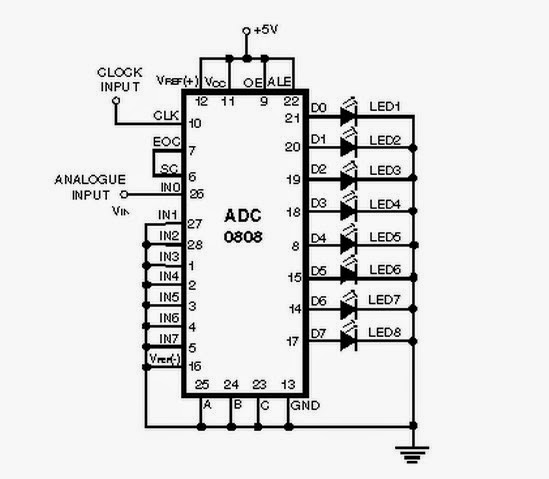## Friday, May 1, 2015

### Simple Circuit Schematic Analog to Digital Converter using ADC 0808 IC

Eltronicschool. - When we work with computer, computer will only process the discrete signal or digital signal. So, when we have continuous signal or analog signal it is need to convert in to digital signal to process by computer.

We need analog to digital converter circuit when we want work from analog signal to digital signal like in figure 1 below. This analog to digital converter circuit using ADC 0808 IC with 8 bits output.The ADC 0808 is an 8-bit A-to-D converter, having data lines D0-D7. It works on the principle of successive approximation. It has a total of eight analogue input channels, out of which any one can be selected using address lines A, B and C. Here, in this case, input channel IN0 is selected by grounding A, B and C address lines.

Circuit SchematicFigure 1. Schematic Analog to Digital Converter using ADC 0808 IC(Source: electronic-circuits-diagrams)

Description

Circuit schematic like in figure 1 above is Simple Circuit Schematic Analog to Digital Converter using ADC 0808 IC. The ADC 0808 IC requires clock signal of typically 550 kHz, which can be easily derived from an astable multivibrator constructed using 7404 inverter gates. In order to visualise the digital output, the row of eight LEDs (LED1 through LED8) have been used, wherein each LED is connected to respective data lines D0 through D7. Since ADC works in the continuous mode, it displays digital output as soon as analogue input is applied. The decimal equivalent digital output value D for a given analogue input voltage Vin can be calculated from the relationship. Read more the description using link source below.